# SAS a00-215 practice test

## SAS 9.4 Programming Fundamentals Exam Exam

##### Question 1

CORRECT TEXT
Given the following DATA step: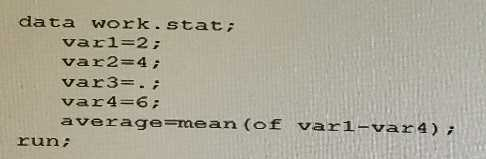What is the value of average?

3

Discussions

##### Question 2

Given the code shown below: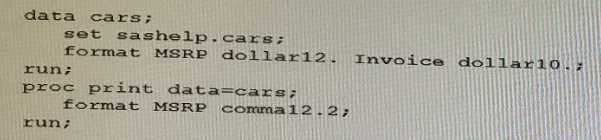What will be the format for MSRP in the RPOC PRINT output?

• A. There is a syntax error in the FORMAT statement in the PROC PRINT step.
• B. Comma12. 2
• C. Dollar10.
• D. Dollar12.

D

Discussions

##### Question 3

The sashelp. class data set has 19 observations.
Given the frequency information about the Age, shown below: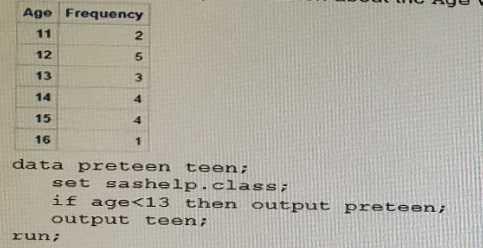How many observations are written to output data set when the following code is submitted?

• A. preteen will have 7 observations and teen will have 19 observations
• B. preteen will have 7 observations and teen will have 12 observations
• C. preteen will have 26 observations and teen will have 31 observations
• D. preteen will have 10 observations and teen will have 9 observations

C

Discussions

##### Question 4

Given the PROC PRINT report of the INVEST data set shown below: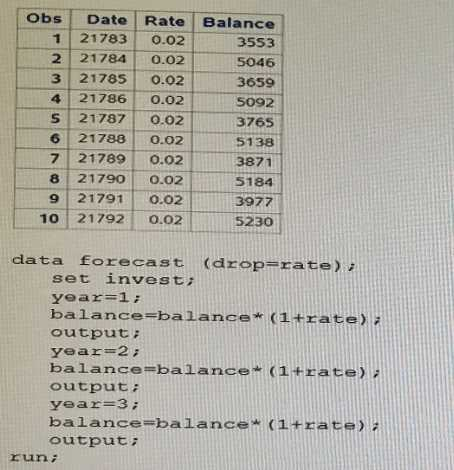How many observations are in the FORCAST data set after this program executes?

• A. 30
• B. 10
• C. 0
• D. 20

A

Discussions

##### Question 5

Given the input data set WORK.RUN: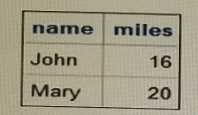Given the following DATA step: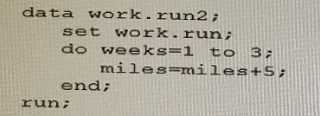What is the correct output data set WORK.RUN2?
A)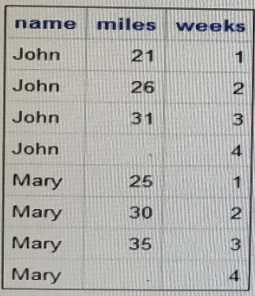B)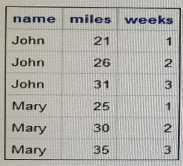C)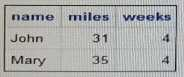D)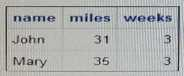• D. Option

D

Discussions

##### Question 6

Given the program shown below: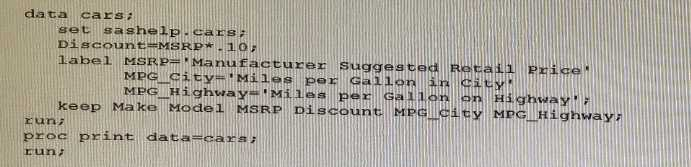Given the partial PROC PRINT report below: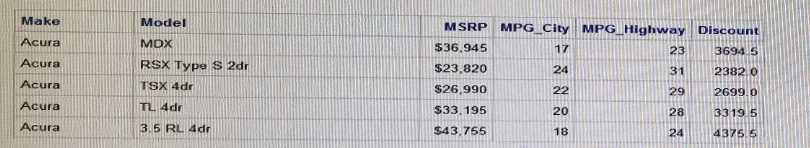Why are the labels for msbp, MPG_city, and MPG_Highway NOT displaying in the PROC PRINT
report^

• A. You must use the LABEL option on the PROC PRINT statement
• B. You must put the LABEL statement in the PROC PRINT step
• C. You must put the LABEL statement after the KEEP statement In the DATA stop
• D. You must use a single LABEL statement for each variable.

A

Discussions

##### Question 7

Which code uses the correct syntax to conditionally create the two variables age-Cat and account?

• A. if age<13 do than; age_Cat= ' Pre-teen '; ,-account='No Social Media;' and;
• B. if age<13 then do; age_cat=' Pre-teen' account='No social Media' Allowed;' and; if age<13 then age_Cat=;Pre-teen' account='No Social Media Allowed';
• D. if aga<13 do; age_Cat-'Pre-teen'; account='No Social Media Allowed end;

B

Discussions

##### Question 8

The SAS log of a submitted DATA step is shown below:
Which action resolve the error messages?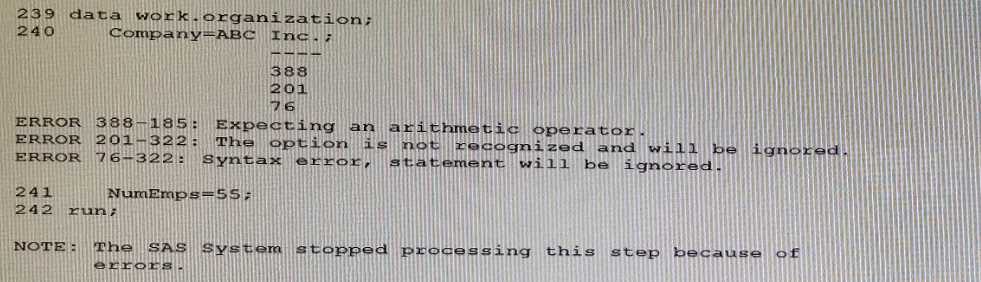• A. Remove the period after the value of Inc.
• B. Enclose the value of ABC Inc . in quotation marks.
• C. Add a LENGTH statement to declare the company variable as character
• D. Enclose the value of ABC Inc. in parentheses.

A

Discussions

##### Question 9

Given the following code: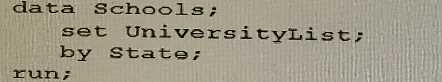Which variables are created with the BY statement?

• A. State. First and State. Last
• B. First. State and Last. State
• C. First_State and Last_State
• D. First. and Last.

C

Discussions

##### Question 10

Which iterative DO statement is invalid?

• A. Do 100 to 1200 by 100;
• B. Do num = 1.1 to 1.9 by 0.1;
• C. Do year = 2000 to 2016 by 2;
• D. Do reverse = 10 to 1 by -1;